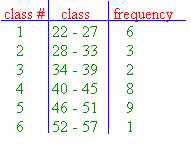### CFA Practice Question

There are 985 practice questions for this topic.

### CFA Practice Question

For the grouped frequency distribution shown below, which of the following is true?A. The minimum data value is 22 and the maximum data value is 57.
B. The number of scores is 29.
C. The class mark for class #1 is 25.

The only true statement is that there are 29 scores. You cannot tell the maximum or minimum from observing a grouped frequency distribution. The class mark for class #1 is 24.5.

User Comment
doreen what is class mark?
wuyi Class Mark: The average of the values of the CLASS LIMITS for a given class. It's also called a midvalue or central value.
wuyi Class #1: 22,23,24,25,26,27. The average value = (24+25)/2=24.5
wuyi To correct:
The lowest limit = 22
The highest limit = 27
Class Mark = (22+27)/2 = 24.5
surob Why the minimum and maximum data value are not right? Can someone explain please?
CFAenlistee Because class 1 can contain 6 scores of 23. So theroticially, no one score 22 points ;)
Bibhu Surob, the question asks minimum and maximum DATA value which could be any of 22,23,24 etc( for min) and 52,53..57( for max). Not necessarily it will be 22 or 57 as min and max values. The grouped frencies distribution has been presented in the chart.
cp24 The lower limit for class 1 is actually 21.5, so the minimum data value can be <22. Same logic for 57.
mynotes Agree with Bibhu and "Correct answer Explanation". For example in class #1, 22 to 27 can be valid elements, as given in column 2. However we do not know what element(s) are actually existed there in the class 1. Even though class #1's frequency is 6, element could be 24 only (repeating 6 times) or any other in the range 22 to 27, that total 6 times. Same is true for the last class in the table.